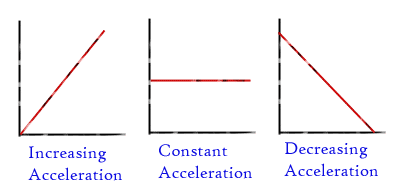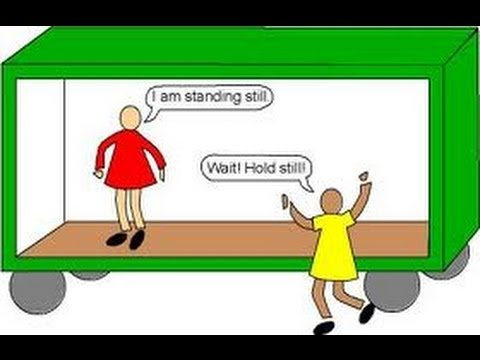# Frames of Reference

## Inertial frame and Non- Inertial Frame of Reference

In this topic we will discuss the idea of reference frame and learn if the laws of physics remain unchanged when we change the frame of our reference while studying or observing certain phenomenon. Albert Einstein famously said that imagination is more important than knowledge and you will need your imagination to understand this topic better. We will also be studying about inertial reference frames and non-inertial reference frames and how are they different from each other. You will need to imagine things like a person running on a parallel road to a moving train. When you relate the concept to the real-world entities it is easier to understand and lasts forever.

Let us go through some basic definition before jumping to the topic.

Velocity:

Velocity is a vector quantity which has both magnitude and direction whereas speed is a scalar quantity which has only the magnitude. To put this in simple terms, velocity is the speed at which an object moves in a particular direction, such as the speed of a bike traveling north on a major freeway, or the speed a rocket moves as it launches into space. The displacement of an object in a certain amount of time means velocity and that velocity has units of distance divided by time, such as miles per hour or kilometers per hour or meters per second. The dimension of velocity is given by L T-1. The change in position divided by the time of travel is said to be average velocity.

v = d / t
Where, v is the velocity
t is the time taken by the object.

Why does velocity even matters

Velocity measures the motion of an object that starts moving from one place and starts heading towards another place. When we put it in the other way, you use measures of velocity to decide how quickly you or any object in motion will arrive at a destination from a given from the starting location.

Measures of velocity provide you to create timetables for travel and plan your schedule accordingly. For example, if a train leaves ABC Station in Bangalore at 6 p.m. and you know the velocity at which the train is moving the other station, you can predict when it will reach the destination station.

Acceleration:Acceleration is the name we give to any method where the velocity changes. Considering velocity is a speed and a direction, there are only a couple of ways for you to accelerate: change your direction or change your speed or change both.

If you’re not changing your speed when you start moving and you’re not changing your direction when traveling, then you simply cannot be accelerating no matter how swiftly you are going. So, a car moving with constant velocity at 850 km per hour along a straight road has zero acceleration, even though the car is moving really fast considering the velocity is not changing. When the car quickly comes to a stop, it will have acceleration since it’s slowing down.

In everyday communication, people use the word deceleration to describe slowing down. But in physics, we use the single word acceleration to mean any change in velocity, whether it be speeding up, changing direction or slowing down.

Relative motion:

Relative motion is just a form of saying that sometimes various people will say distinctive things about the motion of the same object.This is not because one is wrong, but because they are using distinctive frames of reference.
The best way to understand this is possible is to look at some cases.
In all of the following instance, neglect air resistance.

Example 1:

Let us say I am standing on the back of a pickup truck such that they are motionless, and I am throwing oranges forwards. I know that I can throw an orange at exactly 12m/s every time.

• • If a person were standing on the footpath, how fast would she say the oranges are moving?

• • Considering she will see them exactly the same way as me i.e., we are both in the same reference frame (We will discuss reference frame in the upcoming section), she will say 12m/s.

• • Now the truck starts to move forwards at 18m/s. I am still throwing oranges forwards, correctly the same as I was throwing them before, at 12m/s.

• • If I am really not aware of what’s going on around me such that like the point that I am standing in the back of a moving truck, how quickly would I say the oranges are moving?

• • Still, they are moving 12m/s, relative to me, I can only make an orange move apart from me at 12m/s, so that is how fast I measure the orange moving away from me.

• • How quickly does my friend on the sidewalk say the orange is moving?

• • Well, even before I throw it, she will say that the orange is moving at 18m/s such that the speed of everything on the truck.

• • When I have thrown the orange in the front, adding more velocity to it, she will say it is going at (18m/s + 12m/s) 30m/s.

• • Now I turn around and start throwing the same oranges from the rear of the truck, that is backward.

• • I will, however, say that my oranges are moving at 12m/s for me because from the way I am looking at it, that’s how fast the orange is moving. The only thing I might say that is distinctive is that it is -12m/s because even I should be able to see they are going in the opposite direction now.

• • The friend of mine on the footpath will say that the orange is moving at 18m/s + -12m/s = 5m/s.

• In each of the above cases, we are particularly talking about 2 distinctive people having 2 distinctive frames of reference while measuring the relative velocity of an object.

The frame of reference: When we stand on the ground, that is our frame of reference. Anything that you observe, view, or measure will be compared to the reference point of the ground. If you are standing in the back of a moving truck, the truck is now in your frame of reference and everything will be measured compared to it.

Relative velocity, In the above cases, each person was determining the velocity of the oranges relative to which is compared to the frame of reference that you are standing in. Relative to a person standing on the footpath, the orange may be moving at 8 m/s, while for a person in the frame of reference of the truck, the orange is moving at 12 m/s relative to the person.

Example 2:

If you are sitting at your desk, how fast are you moving?

If it is relative to the ground, then there is no moment 'Zero'. You are not moving relative to the frame of reference of the ground.

If it is relative to the sun, then the moment is equal to 2.97e4 m/s. That’s a huge difference, but considering the Earth is orbiting (Revolving) the sun at this rate, an observer standing on the sun would say that you are moving at 2.97e4 m/s.
Both these answers are correct in their own reference of frames.

There are two types of frames of reference:

• 1. Inertial frame of reference

• 2. Non- Inertial frame of reference

• Newton's law holds true for an inertial frame of reference. That indicates if no external (outside) force is acting on a body it will remain at rest or remain in uniform (same) motion. Assume an object is kept on the surface of the earth, for a person viewing it from earth the object is at rest while for a person viewing it from the moon it is in motion, so which is my inertial frame in this case. Actually, the inertial frame is relative that is first, we consider a reference frame to be the inertial frame of reference. In a more general definition of an inertial frame would be Inertial frame is stationary or moves with constant velocity with respect to the expected inertial reference frame.

Now let us determine a non-inertial frame as a frame which is accelerated with regard to the assumed inertial frame of reference. For these frames, Newton's law will not hold true. So, in the above instance if I consider the earth to be an inertial reference frame whereas the moon becomes a non-inertial reference frame this is an accelerated motion with respect to earth. We need to create a pseudo force if we want to make Newton's law.

Answer the following questions:

• 1. Define the term velocity and acceleration?

• 2. What is relative motion and explain with an example?

• 3. Define the term frame of reference?

• 3. Explain Inertial frame of reference and Non- Inertial frame of reference with an example?

• Fill in the blanks:

• 1. The two types of frames of reference are __________ and _____________.

• (Ans: Inertial frame of reference and Non- Inertial frame of reference)
• 2. The dimension of velocity is given by ____ (Ans: L T-1 )

• 3. Newton's law holds true for _________ of reference. (Ans: an inertial frame)

• 4. Velocity is a vector quantity which has both ______ and _______. (Ans: magnitude and direction)